# Category Archives: Maths Challenges

## Times tables finger trick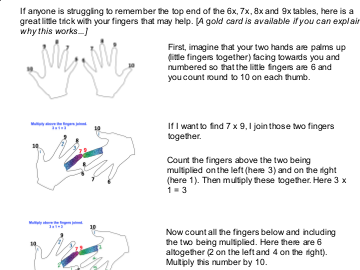View post

## Linear sequences challenge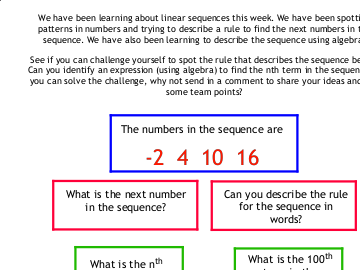View post

Posted in Maths, Maths Challenges, pupils, Year 6 | 2 Comments

## If you want to know more about trigonometry…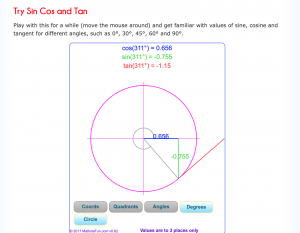Trigonometry is a really interesting branch of mathematics which is all about triangles and their angles.
If you are lucky enough to have a scientific calculator, you could experiment with it to find out about the relationships between the length of different sides of triangles (and different types of triangles) and the sines, cosines and tangents of all the angles.

e.g. If I had an equilateral triangle, all the sides would be equal (let’s say they are all 6 cm). In this case, the cosine of the base angles would be found by dividing half of the baseline (= 3 cm) by the (hypotenuse) side that we know is 6 cm. So we have Cosine of the base angle = 3/6 = 0.5. So inverse Cosine 0.5 is the angle at the base of the triangle… Look it up to see what you get…

There is also a useful site available on ‘Maths is fun’ which will introduce you to the ideas if you want to look further… Click on the image above to follow the link and play with the angles to find out more.

## Investigating isosceles triangles 2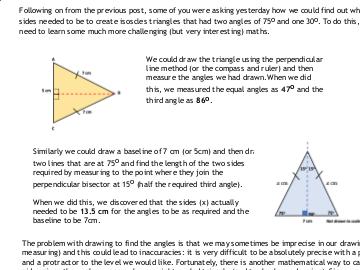View post

## Countdown – fractions game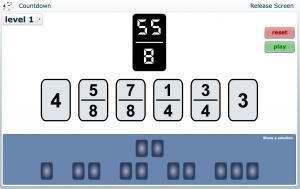Here is the other NRICH Countdown game we tried last week. There are several levels to this game which do get harder. We were managing Levels 1 and 2 pretty well on Friday. Maybe we could try out Level 3? See what you think of it.

## Countdown game – numbers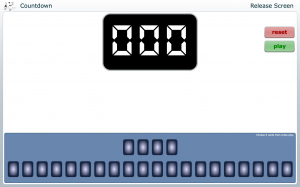Here is the link to the NRICH countdown game we played last week.

Click on the image to go to the online game.

This is a fun way to practise your mental maths and apply your number skills.

## Number lines in disguise

This is another game we tried that you may want to play at home. The idea is to find the missing number on the number line. You can play at several different levels. Start at level 1 and see how far you can get. Be warned! It goes into fractions sometimes so can get rather challenging! Click on the number line to connect to the game.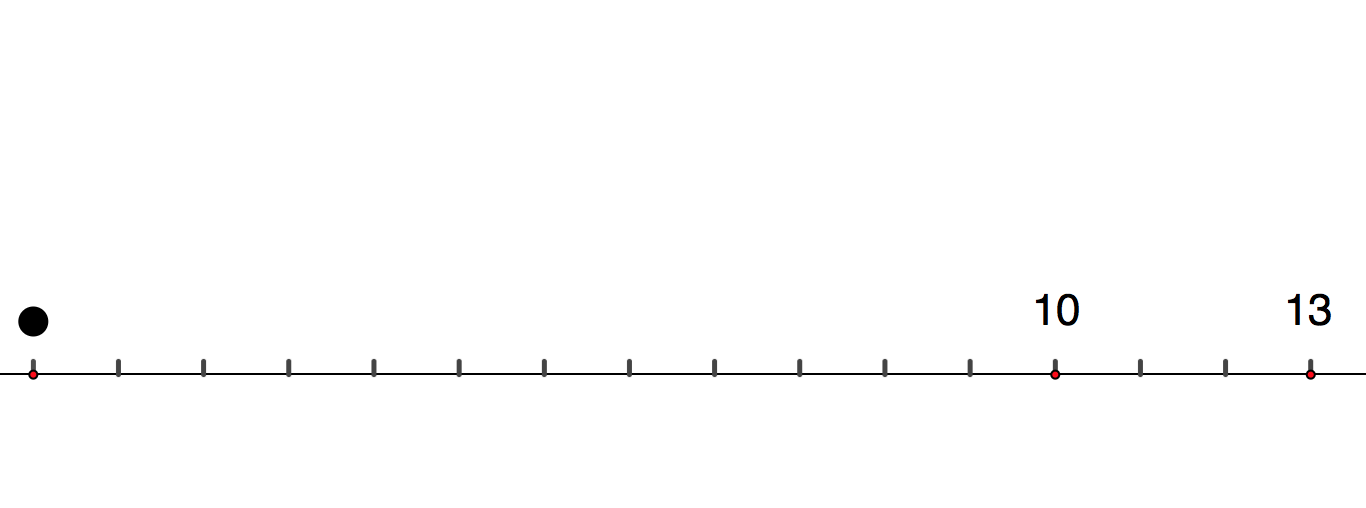## Update on Paper folding polygon challenge

We looked at this problem again today and this time tried to investigate the maximum number of sides on a polygon we could create using one fold starting from paper that was different initial polygons.

With one fold, we found the that the biggest polygons we could make were as below:

Starting with a triangle, one fold produced at most a polygon with 7 sides (heptagon)
With a quadilateral (investigated last time), we got a maximum of 9 sides.
With a pentagon, one fold produced a maximum of 11 sides,
Heptagons folded once produced a maximum of 13 sides,
etc.

We think we have a definite pattern now and think we have a general expresssion to describe the largest polygon that can be made from a piece of paper with x sides using n folds now is:
Maximum number of sides in folded polygon = x(n+1) +1

Well done for trying so many good ideas Y6 to investigate this.

## Paper-folding maths problem

We had a fun maths lesson on Friday which has left us all thinking!

We started with oblong pieces of paper (A5) and the challenge was to see how many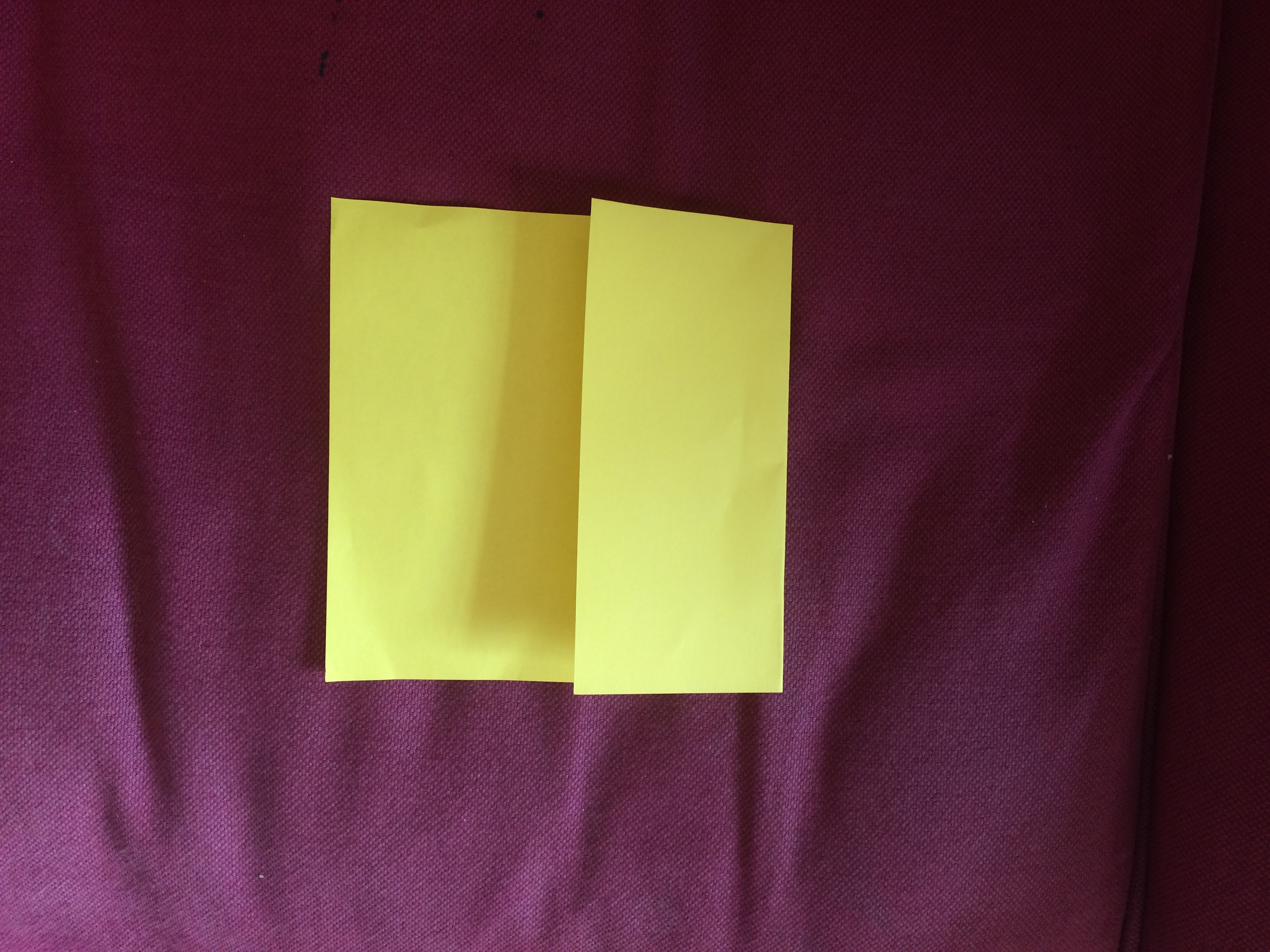different polygons we could make using one fold only. We then had to explore the properties of the different shapes and look for symmetry, regularity, etc. We discovered that we could not make a triangle with only one fold, but we could make a variety of quadrilaterals (discussed square (regular quadrilateral), trapezium, creating different parallelograms, etc.).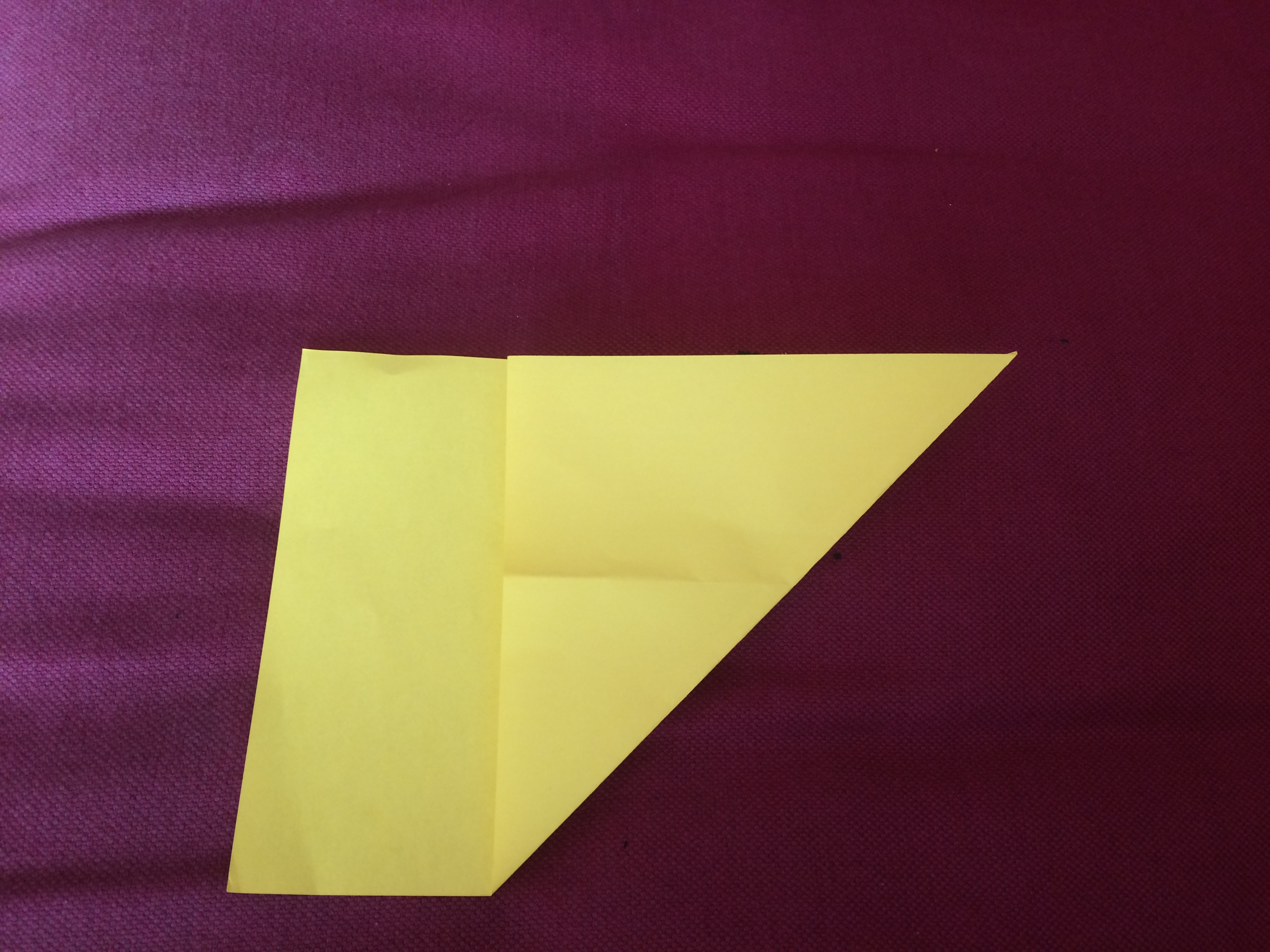Once we started looking at higher order polygons, we discovered we could make shapes up to nonagons but none were regular and only the shapes with odd numbers of sides (pentagon, heptagon, nonagon) could be created to have a line of symmetry with one fold.

Next someone suggested seeing how many different polygons they could make with two folds. Some people insisted they could make a tetradecagon (14), but others thought they could only make 13 (triskaidecagon). We are not entirely sure whether we are folding accurately so it would be good to try this again and confirm which is correct.

We decided then that we would spend the weekend investigating this further to see if there is a pattern that we can come up with to predict the maximum number of sides of polygons that can be made with a given number of folds and whether there is a mathematical explanation for this. We are sure there must be but we think we might need help to find out. So far, we think we have a sequence of 1 fold (max 9 side), 2 folds (max 13 sides), 3 folds (17 sides) which looks like there could be a pattern developing but it is getting harder and harder to be sure we have folded correctly which is why we think it would be good to see what other people think. We are wondering whether the increase in 4 each time has also got something to do with the number of sides in our initial shape (the oblong paper). Maybe we could investigate this further using a different polygon to start us off?

I have asked our friends in Year 6 at Long Itchington to see if they can help and am also asking some other people who love maths problems. I’ll let you know if we find out! If you discover anything more, it would be great to add a comment. There is a gold card for anyone coming up with ideas that they can explain to me clearly! We want to crack this before the end of term!

Posted in Maths, Maths Challenges, pupils, Year 6 | 2 Comments

We’ve been trying out some practical fractions challenges where we had to work out the fractions of different shapes when we had limited information. We investigated the shapes to discover which ones fitted exactly inside other ones. Then we used this information to work out the fraction each of the shapes were as a fraction of the blue square (the largest shape). Only the orange, red and yellow triangles fitted exactly inside the blue square. The other shapes we had to work out by comparing them to the triangles. We eventually discovered that the other shapes were the following fractions of the blue square:
Green square = 1/2                     Orange triangle = 1/4
Red triangle = red square = pink triangle = 1/8
Yellow triangle = 1/32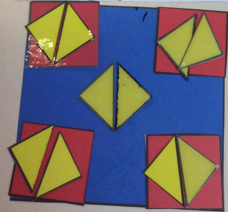Then we set ourselves some more challenges. We had to make some patterns using the different shapes and then work out the fractions of the whole shape each colour represented. The pictures below show some of our challenges. e.g. On this one, there are 10 yellow triangles so we know that yellow is 10/32 = 5/16 of the square. But, the yellow covers some of the red, so we need to work out the red base and then subtract the yellow to find the fraction that is red in the final shape. Then we could finally work out the fraction that is blue… Not as easy as it sounds!

These were tricky for different reasons but some of them are especially tricky because the pattern makes us think we have one fraction for a colour whereas the calculations show something different…. We found out that we always needed to check that the fractions of all the parts added up to make one whole one…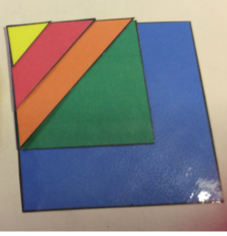Using the information above, we worked out the fractions of all the colours in this ‘rainbow’ square made by laying other shapes on top of each other on top of the blue square..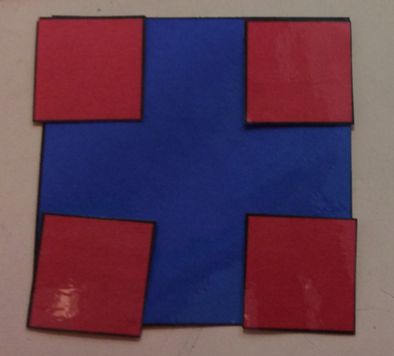This was another tricky one: We needed to check the information we started with very carefully to solve this one…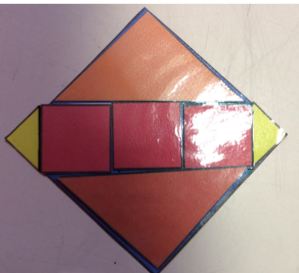This one was especially tricky as the layout suggests that there are only three colours in the shape, but we discovered that there were, in fact, four… We ended up finding the fraction of the (almost) hidden colour too…